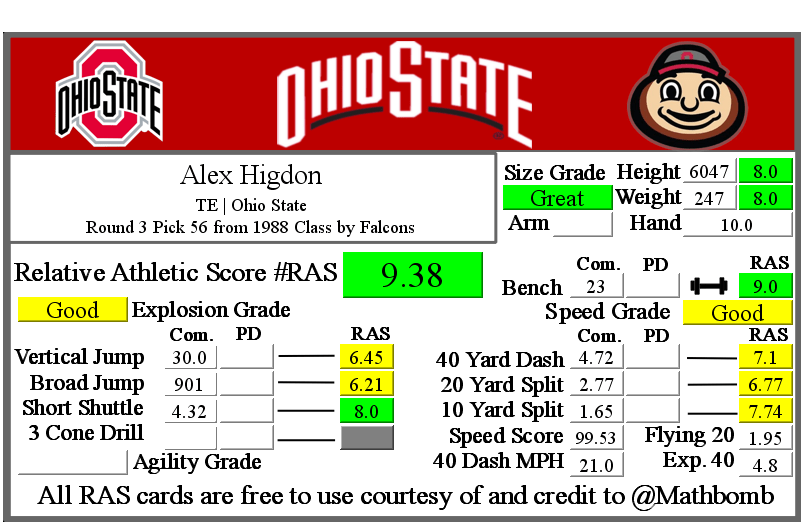# Alex Higdon RAS

### Alex Higdon RASAlex Higdon was drafted by Falcons with pick 56 in round 3 in the 1988 NFL Draft out of Ohio State.

He recorded a Relative Athletic Score of 9.38, out of a possible 10.0. RAS is a composite metric on a 0 to 10 scale based on the average of all of the percentile for each of the metrics the player completed either at the Combine or pro day.

He had a recorded height of 6047 that season, recorded as XYYZ where X is feet, YY is inches, and Z is eighths of an inch. That correlates to 6 feet, 4 and 7/8 of an inch or 76.875 inches, or 195.2625 centimeters. This correlates to a 8.0 score out of 10.0.

He recorded a weight of 247 in pounds, which is approximately 112 kilograms. This correlates to a 8.0 score out of 10.0.

Based on his weight, he has a projected 40 yard dash time of 4.8. This is calculated by taking 0.00554 multiplied by his weight and then adding 3.433.
At the Combine, he recorded a 40 yard dash of 4.72 seconds. This was a difference of -0.08 seconds from his projected time. This forty time correlates to a 7.1 score out of 10.0.

Using Bill Barnwell’s calculation, this Combine 40 time gave him a Speed Score of 99.53.

The time traveled between the 20 and 40 yard lines is known as the Flying Twenty. As the distance is also known, we can calculate the player’s speed over that distance. The time he traveled the last twenty yards at the Combine was 1.95 seconds. Over 20 yards, we can calculate his speed in yards per second to 10.26. Taking into account the distance in feet (60 feet), we can calculate his speed in feet per second to 30.77. Breaking it down further, we can calculate his speed in inches per second to 369.23. Knowing the feet per second of 30.77, we can calculate the approximate miles per hour by multiplying that value by 0.681818 to give us a calculated MPH of 21.0 in the last 20 yards of his run.

At the Combine, he recorded a 20 yard split of 2.77 seconds. This correlates to a 6.77 score out of 10.0.

We can calculate the speed traveled over the second ten yards of the 40 yard dash easily, as the distance and time are both known. The time he traveled the second ten yards at the Combine was 1.12 seconds. Over 10 yards, we can calculate his speed in yards per second to 8.93. Taking into account the distance in feet (30 feet), we can calculate his speed in feet per second to 26.79. Breaking it down further, we can calculate his speed in inches per second to 321.43. Knowing the feet per second of 26.79, we can calculate the approximate miles per hour by multiplying that value by 0.681818 to give us a calculated MPH of 18.3 in the second ten yards of his run.

At the Combine, he recorded a 10 yard split of 1.65 seconds. This correlates to a 7.74 score out of 10.0 that when multiplied by ten roughly corresponds to percentile.

The time he traveled the first ten yards at the Combine was 1.65 seconds. Over 10 yards, we can calculate his speed in yards per second to 6.0. Taking into account the distance in feet (30 feet), we can calculate his speed in feet per second to 18.0. Breaking it down further, we can calculate his speed in inches per second to 218.0. Knowing the feet per second of 18.0, we can calculate the approximate miles per hour by multiplying that value by 0.681818 to give us a calculated MPH of 12.3 in the first ten yards of his run.

At the Combine, he recorded a bench press of 23 repetitions of 225 pounds. This correlates to a 9.0 score out of 10.0.

At the Combine, he recorded a vertical jump of 30.0 inches. This correlates to a 6.45 score out of 10.0.

At the Combine, he recorded a broad jump of 901, which is recorded as FII or FFII . where F is feet and I is inches. This correlates to a 6.21 score out of 10.0 that when multiplied by ten roughly corresponds to percentile.

At the Combine, he recorded a 5-10-5 or 20 yard short shuttle of 4.32 seconds. This correlates to a 8.0 score out of 10.0.

This player did not have a recorded 3 cone L drill for the Combine in the RAS database.

This player did not record any measurements at their pro day that we were able to find. If they recorded Combine measurements, they stood on them.

This site uses Akismet to reduce spam. Learn how your comment data is processed.